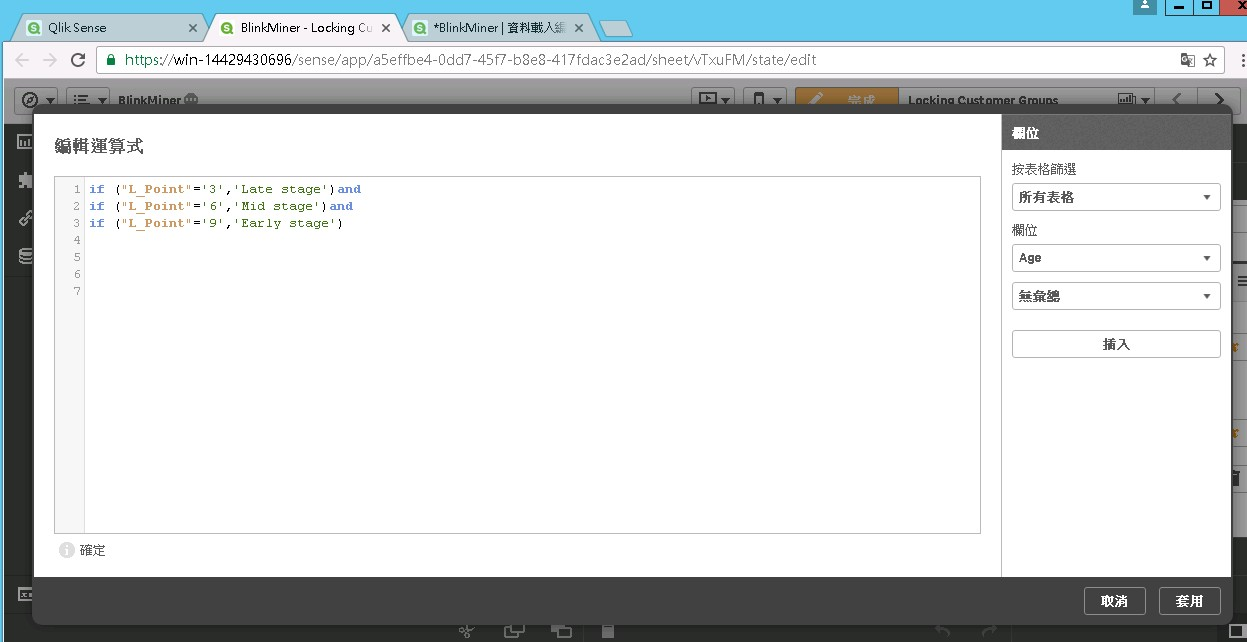# New to Qlik Sense

Discussion board where members can get started with Qlik Sense.

Announcements
Qlik® Product Spotlight: Discover what’s possible. Get more from our products.
See for yourself. Register today.Not applicable

## To write IF statement in load script

I try the IF statement

if ("L_Point"='3','Late stage')　and

if ("L_Point"='6','Mid stage')　and

if ("L_Point"='9','Early stage')

but it's not work?! why? what the correct IF statement?1 Solution

Accepted Solutions
Valued Contributor II

## Re: To write IF statement in load script

Try:

if ("L_Point"='3','Late stage',

if ("L_Point"='6','Mid stage',

if ("L_Point"='9','Early stage')))

Please ekskuse my Norglish and Swenglish typos.
3 Replies
Valued Contributor II

## Re: To write IF statement in load script

Try:

if ("L_Point"='3','Late stage',

if ("L_Point"='6','Mid stage',

if ("L_Point"='9','Early stage')))

Please ekskuse my Norglish and Swenglish typos.
Valued Contributor

## Re: To write IF statement in load script

Try

if(L_Point='3','Late stage', if(L_Point='6','Mid stage', if(L_Point='9','Early stage')))

This doesn't dictate what to do if the L_Point isn't 3, 6 or 9 so I am assuming it can't be any other number. If it can you will want to add something to the expression for example:

if(L_Point='3','Late stage', if(L_Point='6','Mid stage', if(L_Point='9','Early stage', Null())))

or

if(L_Point='3','Late stage', if(L_Point='6','Mid stage', if(L_Point='9','Early stage', L_Point)))MVP

## Re: To write IF statement in load script

pick(match(L_Point,      '3',' '6', '9'),

'Late stage', 'Mid stage', 'Early stage')

Community Browser